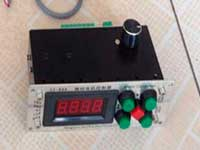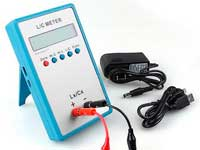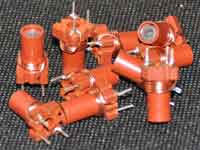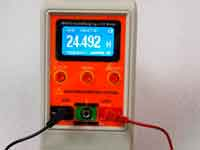LC circuit calculation - 3.3 out of 5 based on 3 votes
Rating 3.33 (3 Votes)

LC circuit calculationIn "LC circuit" sheet the Coil32 computes the values of capacitance, inductance, and frequency for a resonance oscillating circuit. The calculation is made by the Thomson's formula, derived in 1853, and which is all we know of the school course of physics:

where:• f - frequency (Hz);
• L - inductance (H);
• C - capacitance (F);

The program allows you to calculate:

• The resonant frequency of the LC-circuit at a known capacitance and inductance• The LC-tank capacitance at a known resonant frequency and inductance
• The inductance of an LC-circuit for a known capacitance and the resonant frequency

Such a calculation is also implemented in the online calculator of the LC-circuit.

In the "Coil" sheet for single-layer coils, the program generates additional results for parallel LC-circuit:

• The capacitance at the resonant frequency.
• The characteristic impedance of the circuit:• The equivalent resistance of the circuit:By default, these calculations are not displayed. To enable output additional calculations in the output text box you need to click the corresponding button in the toolbar above it.

Calculations are correct of course for an unloaded circuit.
Q-factor contour of the capacitor is not taken into account.
The self-capacitance of the coil is not taken into account, so these calculations are meaningful at frequencies much smaller than the self-resonance frequency of the coil.

Сomments from anonymous guests are enabled with moderation.

Coil Winding MachineUS \$187.53
The system consists of individual components including a controller, a stepping motor, a foot switch, power adapter and output shaft. This design makes it very easy for you to integrate the system into your own project.

LC Meter LC200AUS \$37.99
Measuring Capacitance Range:
0.01 pF - 100mF
Measuring Inductance Range:
0.001 uH - 100H
Measurement accuracy:1%.

RF InductorsUS \$6.43
Variable Ferrite Core Inductor coil set 3.5Turns 25-100MHZ

Auto Ranging LCR MeterUS \$63.07
Meter can measure 0.00pF to 100.00mF capacitance, 0.000uH to 100.00H inductance and 0.00? to 10.000M? resistance. Very suitable for small value capacitor and inductor measurement.